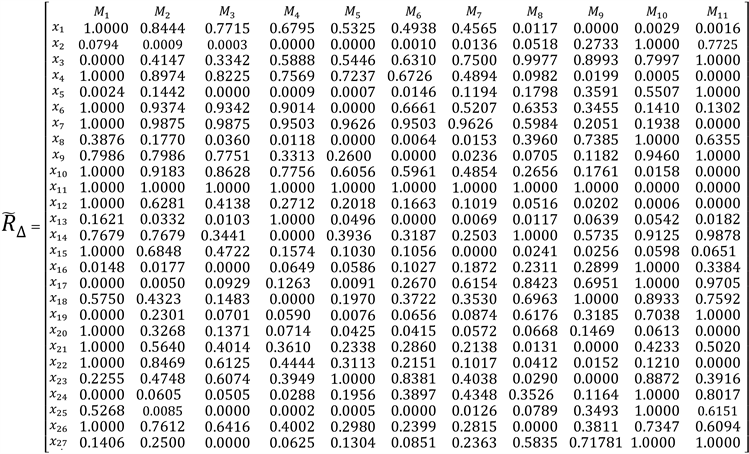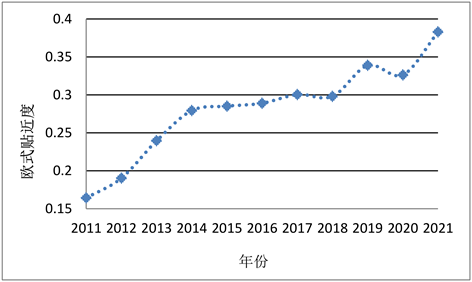#### 期刊菜单

Evaluation and Research on the Development Index of Cold Chain Logistics of Aquatic Products in Shandong Province

Abstract: In order to fully understand the comprehensive development level of cold chain logistics of aquatic products in Shandong Province, based on the research on cold chain logistics and cold chain logistics of aquatic products, this paper takes the cold chain logistics of aquatic products in Shandong Province as the research object. The construction of four first level indicators and 27 second level indicator systems including aquatic product cold chain logistics supports environment, production and processing capacity, economic and trade level and scientific and technological development level. The weights of each index are calculated by using the entropy weight method. .From 2011 to 2021, the Euclidean closeness degree was calculated by fuzzy matter-element method. The development level of cold chain logistics of aquatic products in Shandong Province is comprehensively evaluated according to the closeness measurement value. The results show that the overall development level of cold chain logistics of aquatic products in Shandong Province is on the rise year by year, but there is a slight decline in some years. Finally, according to the evaluation results, starting from the situation of Shandong Province, combined with the basis of economic development and market demand, relevant countermeasures and suggestions for its development direction are given, in order to promote the vigorous development of the cold chain logistics industry of aquatic products in Shandong Province.

1. 引言

2. 研究综述

3. 山东省水产品冷链物流发展评价指标体系Table 1. Evaluation index system of cold chain logistics development level of aquatic products in Shandong Province

4. 山东省水产品冷链物流发展评价模型方法

4.1. 模糊物元法确定欧式贴近度

1) 复合模糊物元。对于m个事物包含的n维复合物元，将构成复合物元矩阵 ${R}_{mn}$ 记作：

${R}_{mn}=\left[\begin{array}{ccccc}& {M}_{1}& {M}_{2}& \cdots & {M}_{m}\\ {C}_{1}& {x}_{11}& {x}_{21}& \cdots & {x}_{m1}\\ {C}_{2}& {x}_{12}& {x}_{22}& \cdots & {x}_{m2}\\ ⋮& ⋮& ⋮& \ddots & ⋮\\ {C}_{n}& {x}_{1n}& {x}_{2n}& \cdots & {x}_{mn}\end{array}\right]$ (3.1)

${R}_{mn}$ ：m个事物n维复合模糊物元；

${M}_{i}$ ：第i个研究事物， $i=1,2,\cdots ,m$

${C}_{j}$ ：第j项特征， $j=1,2,\cdots ,n$

${X}_{ij}$ ：模糊量值。

2) 从优隶属度模糊物元。根据不同的指标具有不同的属性，采用相对应的类型计算最优型量值，根据计算的最优型量值构建从优隶属度模糊物元 ${\stackrel{˜}{R}}_{mn}$，具体公式如下：

${\stackrel{˜}{R}}_{mn}=\left[\begin{array}{ccccc}& {M}_{1}& {M}_{2}& \cdots & {M}_{m}\\ {C}_{1}& {u}_{11}& {u}_{21}& \cdots & {u}_{m1}\\ {C}_{2}& {u}_{12}& {u}_{22}& \cdots & {u}_{m2}\\ ⋮& ⋮& ⋮& \ddots & ⋮\\ {C}_{n}& {u}_{1n}& {u}_{2n}& \cdots & {u}_{mn}\end{array}\right]$ (3.2)

${u}_{ij}=\frac{{x}_{ij}-\mathrm{min}{x}_{ij}}{\mathrm{max}{x}_{ij}-\mathrm{min}{x}_{ij}}$ (3.3)

${u}_{ij}=\frac{\mathrm{max}{x}_{ij}-{x}_{ij}}{\mathrm{max}{x}_{ij}-\mathrm{min}{x}_{ij}}$ (3.4)

${\stackrel{˜}{R}}_{mn}$ ：m个事物的n维复合模糊物元对应的从优隶属度模糊物元；

${u}_{ij}$ ：第i个事物第j项特征所对应的从优隶属度；

${x}_{ij}$ ：第i个事物第j项特征所对应的模糊量值；

$\mathrm{max}{x}_{ij}$ ：对比特征量值，选取最大值；

$\mathrm{min}{x}_{ij}$ ：对比特征量值，选取最小值。

3) 差平方复合模糊物元。根据步骤2)中所求出的隶属度 ${u}_{ij}$ 减掉1后再平方，构建差平方复合模糊物元 ${\stackrel{˜}{R}}_{\Delta }$，具体公式如下：

${\Delta }_{ij}={\left({u}_{ij}-1\right)}^{2}$ (3.5)

${\stackrel{˜}{R}}_{\Delta }=\left[\begin{array}{ccccc}& {M}_{1}& {M}_{2}& \cdots & {M}_{m}\\ {C}_{1}& {\Delta }_{11}& {\Delta }_{21}& \cdots & {\Delta }_{m1}\\ {C}_{2}& {\Delta }_{12}& {\Delta }_{22}& \cdots & {\Delta }_{m2}\\ ⋮& ⋮& ⋮& \ddots & ⋮\\ {C}_{n}& {\Delta }_{1n}& {\Delta }_{2n}& \cdots & {\Delta }_{mn}\end{array}\right]$ (3.6)

${\stackrel{˜}{R}}_{\Delta }$ ：公式(3.6)中相应的差平方复合模糊物元；

${u}_{ij}$ ：第i个事物第j项特征所对应的从优隶属度；

${x}_{ij}$ ：各模糊物元与最优化模糊物元差的平方。

4.2. 熵权法确定客观权重

1) 计算第j个评价指标中第i个指标值的比重 ${R}_{ij}$

${R}_{ij}=\frac{{u}_{ij}}{{\sum }_{i=1}^{m}{u}_{ij}}$ (3.7)

2) 计算第j个评价指标的熵值 ${e}_{j}$

${e}_{j}=-k{\sum }_{i=1}^{m}{P}_{ij}\ast \mathrm{ln}{p}_{ij}$ (3.8)

3) 计算第j个评价指标权重 ${w}_{j}$

${w}_{j}=\frac{1-{e}_{j}}{{\sum }_{j=1}^{n}1-{e}_{j}}$ (3.9)

4.3. 欧式贴近度复合模糊物元

$\rho {H}_{i}=1-\sqrt{{\sum }_{j=1}^{n}{w}_{j}{\Delta }_{ij}}\text{\hspace{0.17em}}\text{\hspace{0.17em}}\left(i=1,2,\cdots ,m\right)$ (3.10)

${\stackrel{˜}{R}}_{\rho H}=\left[\begin{array}{ccccc}& {M}_{1}& {M}_{2}& \cdots & {M}_{t}\\ \rho {H}_{i}& \rho {H}_{1}& \rho {H}_{2}& \cdots & \rho {H}_{t}\end{array}\right]$ (3.11)

5. 山东省水产品冷链物流发展实证研究

5.1. 数据来源

5.2. 评价指标体系构建

1) 首先，根据公式(3.1)构建复合模糊物元矩阵；其次，依照公式(3.2)、(3.3)、(3.4)计算最大最优型、最小最优型，从而构建从优隶属度复合模糊物元矩阵模型；最后，利用公式(3.5)计算差平方数值，以此为依据构建差平方复合模糊物元 ${\stackrel{˜}{R}}_{\Delta }$，最终结果如下所示(保留小数点后4位)。2) 确定权重系数。把标准化之后的uij代入公式(3.7)、(3.8)及(3.9)中，计算在时间维度分析下影响山东省水产品冷链物流各指标的信息熵值 ${e}_{j}$ 、冗余度 $1-{e}_{j}$ 及指标权重 ${w}_{j}$ (保留小数点后4位)计算结果如表2Table 2. Information entropy, redundancy and index weight of each index in Shandong Province

3) 确定复合模糊物元。将所计算得出的各二级指标权重 ${w}_{j}$${\stackrel{˜}{R}}_{\Delta }$ 中的数值代入公式(3.10)，计算山东省2010~2021年水产品冷链物流发展水平的欧式贴近度，构建欧式贴近度复合模糊物元 ${\stackrel{˜}{R}}_{\rho H}$ (保留小数点后4位)，欧式贴近度随时间的变化趋势如图1所示。

${\stackrel{˜}{R}}_{\rho H}=\left[\begin{array}{c}\text{'}\\ \rho {H}_{i}\end{array}\text{\hspace{0.17em}}\text{ }\text{ }\begin{array}{c}{M}_{1}\\ 0.1646\end{array}\text{\hspace{0.17em}}\text{ }\text{ }\begin{array}{c}{M}_{2}\\ 0.1907\end{array}\text{\hspace{0.17em}}\text{ }\text{ }\begin{array}{c}{M}_{3}\\ 0.2399\end{array}\text{\hspace{0.17em}}\text{ }\text{ }\begin{array}{c}{M}_{4}\\ 0.2796\end{array}\text{\hspace{0.17em}}\text{ }\text{ }\begin{array}{c}{M}_{5}\\ 0.2854\end{array}\text{\hspace{0.17em}}\text{ }\text{ }\begin{array}{c}{M}_{6}\\ 0.2893\end{array}\text{\hspace{0.17em}}\text{ }\text{ }\begin{array}{c}{M}_{7}\\ 0.3007\end{array}\text{\hspace{0.17em}}\text{ }\text{ }\begin{array}{c}{M}_{8}\\ 0.2984\end{array}\text{\hspace{0.17em}}\text{ }\text{ }\begin{array}{c}{M}_{9}\\ 0.3391\end{array}\text{\hspace{0.17em}}\text{ }\text{ }\begin{array}{c}{M}_{10}\\ 0.3267\end{array}\text{\hspace{0.17em}}\text{ }\text{ }\begin{array}{c}{M}_{11}\\ 0.3833\end{array}\right]$Figure 1. The level of cold chain logistics of aquatic products in Shandong Province from 2010 to 2021 is European-style closeness

5.3. 结果分析

6. 对策建议

1) 促进水产品消费水平。目前我国水产品消费量呈逐年上升的趋势，但是不能忽略农村消费市场的重要性，为充分挖掘农村市场潜力，可在完善农村交通状况和提高手机普及率的同时，借助电商平台、短视频平台等方式带动冷冻水产品消费。

2) 构建冷链物流网络。可根据山东省水产品冷链发展基础和市场需求，建立连接水产品产运销各环节的冷链物流网络，实现资源整合，降低市场风险水平，提高市场集中度，达到水产品冷链物流设施设备整合、各环节信息流通、各链条有效对接的目的。

3) 扶持冷链物流企业发展。政府可以鼓励水产品冷链物流企业建立协作关系，加强企业间合作沟通，提高山东省水产品冷链物流效益规模。企业内部应制定相应措施促进产运销有效对接，同时加强与第三方物流的合作，促进山东省水产品冷链物流的协调发展。

4) 加强水产品冷链物流科技发展。山东省省政府和各水产品冷链物流企业，应制定水产品冷链物流政策法规和企业内部规章制度，以促进科技水平发展，并鼓励建设高质量的水产技术推广机构。同时，企业应积极改善冷链物流工作环境，提高高质量、高水平、高素质人才培养机制，增强企业内部水产品技术水平。

7. 结论

  剌美香, 李玉萍. 基于FAHP的山西省农产品冷链物流综合评价[J]. 山西农业科学, 2017, 45(8): 1349-1352+1360.  郭明德, 李红. 农产品冷链物流发展水平评价——基于12典型省(市)数据分析[J]. 商业经济研究, 2019(1): 125-127.  柳航, 刘静, 田琪琛, 袁小雨. 襄阳现代物流业发展水平评估[J]. 轻工科技, 2018, 34(3): 103-104+106.  张巧巧, 周骞, 朱海燕. 水产品冷链物流系统安全性评价研究[J]. 公路与汽运, 2019(6): 42-46+51.  谢清玲. 中国东部地区水产品冷链物流发展水平评价[D]: [硕士学位论文]. 广州: 仲恺农业工程学院, 2020. https://doi.org/10.27700/d.cnki.gzcny.2020.000098  张喜才, 张利庠. 我国农产品冷链物流区域发展水平评价研究[J]. 农业科学, 2018, 8(4): 225-236. https://doi.org/10.12677/HJAS.2018.84036  李伟莹, 于洋. 基于灰色预测模型的我国水产品冷链物流需求预测[J]. 农技服务, 2019, 36(6): 103-105+107.  邓延伟, 邬文兵, 于腾群. 水产品冷链运输绩效评价研究[J]. 山东社会科学, 2014(4): 145-150.  盛文, 聂志刚, 孙彬益. 基于层次分析法的辽宁省水产品电商冷链物流绩效评价[J]. 电子商务, 2020(12): 6-8.  方凯, 钟涨宝, 王厚俊, 贺岚. 基于绿色供应链的我国冷链物流企业效率分析[J]. 农业技术经济, 2014(6): 45-53. https://doi.org/10.13246/j.cnki.jae.2014.06.006